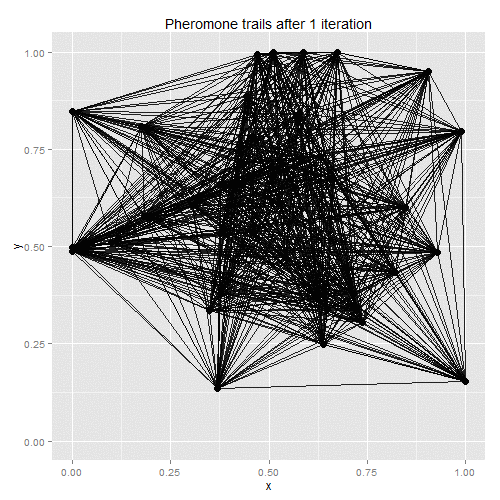Home > Misc

# Ant-colony optimisation

Ant-colony optimisation was developed as a way of finding good solutions to the travelling salesman problem, with the algorithm based on real-life ant behaviour. Some number of artificial ants are sent out to tour the given vertices, with their paths chosen stochastically. At each vertex, ants will favour vertices that are closer and which have the most pheromones along the edge to the current vertex. Once all ants have returned to the starting point, the existing pheromone trails evaporate a little, and new pheromones are deposited along the shortest path taken by any of the ants. On the next iteration, therefore, ants will be more likely to follow the shortest path found on the previous iteration, with the random choices eventually helping to find a solution close to the global optimum.

The process lends itself to gif-making:Though it can fail to converge (at least in a time shorter than my patience) in some edge cases:

R code follows.

``````library(ggplot2)

# Following is needed to avoid sample()'s bizarre behaviour with inputs of length 1:
resample <- function(x, ...) x[sample.int(length(x), ...)]

# Number of times we send out the ants:
num_iter <- 200

# Define some locations:
num_pts <- 40
x <- rnorm(num_pts, mean=0.5, sd=0.25)
y <- rnorm(num_pts, mean=0.5, sd=0.25)
x[which(x>1)] <- 1
x[which(x<0)] <- 0
y[which(y>1)] <- 1
y[which(y<0)] <- 0

# The following are vectors containing either the start or end of each edge.
# They will be used as the input to geom_segment.
x1 <- numeric()
y1 <- numeric()
x2 <- numeric()
y2 <- numeric()
for (i1 in 2:num_pts) {
for (i2 in 1:(i1-1)) {
x1 <- c(x1, x[i1])
y1 <- c(y1, y[i1])
x2 <- c(x2, x[i2])
y2 <- c(y2, y[i2])
}
}

num_ants <- 25
# Some parameters - how quickly the pheromones evaporate, and the exponents used
# when weighting the probabilities for the ants to choose an edge:
evap_rate <- 0.15
pher_exp <- 0.5
inv_dist_exp <- 3

pts <- data.frame(x, y)
# Distances between the points:
dist_matrix <- as.matrix(dist(pts))

# The inverse-distance matrix, and the inverse-distance matrix raised
# to the power defined above:
inv_dist_matrix <- dist_matrix
diag(inv_dist_matrix) <- rep(1, num_pts)
inv_dist_matrix <- 1/inv_dist_matrix
inv_dist_matrix_power <- inv_dist_matrix ^ inv_dist_exp

# ggplot the locations:
base_plot <- ggplot() + geom_point(aes(x, y, size=.5)) + guides(size=F) + xlim(0, 1) + ylim(0, 1)

# Start with the same amount of pheromone trail on every edge.
pher_matrix <- matrix(rep(1, num_pts*num_pts), nrow=num_pts, ncol=num_pts)
diag(pher_matrix) <- 0

nodes <- 1:num_pts

for (i in 1:num_iter) {
print(i)

pher_matrix_power <- pher_matrix ^ pher_exp

start_node <- 1

# Vector which will hold the path length of each ant's tour:
ant_dists <- rep(0, num_ants)

# Matrix to store the order of the vertices visited by each ant:
ant_paths <- matrix(rep(0, num_ants*num_pts), nrow=num_ants, ncol=num_pts)

for (ant in 1:num_ants) {
current_node <- start_node
done_nodes <- current_node

for (node_ct in 2:num_pts) {
nodes_left <- which(!(nodes %in% done_nodes))
wts <- inv_dist_matrix_power[current_node, nodes_left] * pher_matrix_power[current_node, nodes_left]
# The weights don't need to be normalised:
new_node <- resample(nodes_left, 1, prob=wts)
ant_dists[ant] <- ant_dists[ant] + dist_matrix[current_node, new_node]

current_node <- new_node
done_nodes <- c(done_nodes, current_node)
}

# Add the distance from the final node to the start node:
ant_dists[ant] <- ant_dists[ant] + dist_matrix[current_node, start_node]
# Add the latest node to the current ant's path:
ant_paths[ant, ] <- done_nodes
}

# Evaporate pheromones:
pher_matrix <- pher_matrix*(1 - evap_rate)

# Find the shortest path and deposit pheromones along it:
shortest_path <- min(ant_dists)
best_ant <- which(ant_dists==shortest_path)
for (node in 1:(num_pts-1)) {
i1 <- ant_paths[best_ant, node]
i2 <- ant_paths[best_ant, node+1]
pher_matrix[i1, i2] <- pher_matrix[i1, i2] + 1/shortest_path
pher_matrix[i2, i1] <- pher_matrix[i1, i2]
}
i1 <- ant_paths[best_ant, num_pts]
i2 <- ant_paths[best_ant, 1]
pher_matrix[i1, i2] <- pher_matrix[i1, i2] + 1/shortest_path
pher_matrix[i2, i1] <- pher_matrix[i1, i2]

max_pher <- max(pher_matrix)
# Re-normalise the pheromone values:
pher_matrix <- pher_matrix / max_pher

# Plot the trails.  Start by creating the vector to store the
# pheromone level along each edge, which will be used as alpha
# values in the geom_segment.
rel_wts <- numeric()
for (i1 in 2:num_pts) {
for (i2 in 1:(i1-1)) {
rel_wts <- c(rel_wts, pher_matrix[i1, i2])
}
}

plural <- "s"
if(i == 1) { plural <- "" }
title_str <- sprintf("Pheromone trails after %d iteration%s", i, plural)

pher_plot <- base_plot + ggtitle(title_str) +
geom_segment(aes(x=x1, y=y1, xend=x2, yend=y2), alpha=rel_wts)

outfile <- sprintf("images/%04d.png", i)
png(filename=outfile, width=500, height=500, units="px")
print(pher_plot)
dev.off()
}```
```

Home > Misc